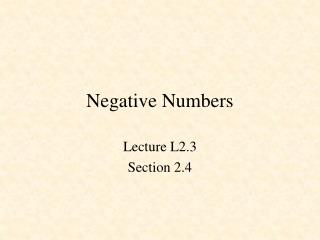DownloadDownload PresentationNegative Numbers

# Negative Numbers

Download Presentation## Negative Numbers

- - - - - - - - - - - - - - - - - - - - - - - - - - - E N D - - - - - - - - - - - - - - - - - - - - - - - - - - -
##### Presentation Transcript

1. Negative Numbers Lecture L2.3 Section 2.4

2. Ignore carry Negative Numbers Subtract by adding 73 -35 38 73 +65 138 10’s complement

3. Negative Numbers 10’s complement: Subtract from 100 Take 9’s complement and add 1 100 -35 65 99 -35 64 +1 65

4. Negative Numbers 2’s complement: Subtract from Take 1’s complement and add 1 100000000 01001101 10110011 11111111 -01001101 10110010 +1 10110011

5. Complement remaining bits Copy all bits to first 1 2’s complement Finding 2’s Complement 0 1 0 1 1 0 0 0 1 0 1 0 1 0 0 0

6. Negative NumberTake 2’s Complement 7510 = 4B16 = 01001011 -7510 = B516 = 10110101 FF -4B B4 +1 B5

7. Negative NumberTake 2’s Complement 110 = 0116 = 00000001 -110 = FF16 = 11111111 12810 = 8016 = 10000000 -12810 = 8016 = 10000000

8. Signed Numbers 4-bit: 8H = -8 to 7H = +7 1000 to 0111 8-bit: 80H = -128 to 7F = +127 16-bit: 8000H = -32,768 to 7FFFH = +32,767 32-bit: 80000000H = -2,147,483,648 to 7FFFFFFFH = +2,147,483,647

9. Questions What is the two’s complement of 00101100? What hex number represents the decimal number -40?